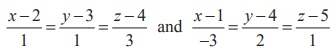Home | | Maths 12th Std | Exercise 6.8: Equation of a plane

# Exercise 6.8: Equation of a plane

Maths Book back answers and solution for Exercise questions - Mathematics : Equation of a plane: Questions with Answer

EXERCISE 6.8

1. Show that the straight lines= (5ˆi +  j - k ) + s(4ˆi + 4 ˆj - k ) and= (8ˆi + 4ˆ j + 5ˆk )+ t (7ˆi + ˆj + 3ˆk ) are coplanar. Find the vector equation of the plane in which they lie.2. Show that the linesare coplanar. Also, find the plane containing these lines.3. If the straight linesare coplanar, find the distinct real values of m.4. If the straight linesare coplanar, find λ and equations of the planes containing these two lines.